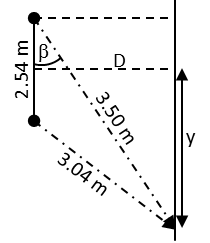# Two loudspeakers are 2.54 m apart. A person stands 3.04 m from one speaker and 3.50 m from the...

## Question:

Two loudspeakers are 2.54 m apart. A person stands 3.04 m from one speaker and 3.50 m from the other. What is the lowest frequency at which destructive interference (sound cancellation) will occur at this point?

## Sound interference

The phenomenon of interference is characteristic of all waves. Thus, two point sources of sound waves can interfere with each other in a similar way to what happens in Young's experiment. In this case we will use the interference minimum equation of Young's experiment:

{eq}d\sin\theta=\left (m+\frac{1}{2} \right )\frac{v}{f} {/eq}

where the wavelength was replaced by the ratio:

{eq}\lambda=\frac{v}{f} {/eq}

where {eq}v\approx343\,\mathrm{m/s} {/eq} is the speed of sound in the air and {eq}f {/eq} the frequency of the sound wave.

First we will calculate the distance that separates the person from the center of the interference pattern and then the distance that separates the speakers from the plane where the interference pattern is being heard.{eq}\mathrm{(3.04\,m)^2=(2.54\,m)^2+(3.50\,m)^2-2(2.54\,m)(3.50\,m)cos\beta\Rightarrow \beta=arccos\left (\frac{(2.54\,m)^2+(3.50\,m)^2-(3.04\,m)^2}{2(2.54\,m)(3.50\,m)} \right )=57.9^\circ}\\ \sin(90^\circ-57.9^\circ)=\frac{y+\frac{2.54\,\mathrm{m}}{2}}{3.50\,\mathrm{m}}\Rightarrow y=\mathrm{(3.50\,m)\sin(90^\circ-57.9^\circ)-\frac{2.54\,m}{2}=0.59\,m}\\ \cos(90^\circ-57.9^\circ)=\frac{D}{3.50\,\mathrm{m}}\Rightarrow D=\mathrm{(3.50\,m)\cos(90^\circ-57.9^\circ)=2.96\,m} {/eq}

Using now the equation corresponding to the interference minimums, we can find the minimum frequency at which destructive interference occurs:

{eq}\left.\begin{matrix} d\sin\theta=\left (m+\frac{1}{2} \right )\frac{v}{f}\\ \sin\theta=\frac{1}{\sqrt{(D/y)^2+1}}\\ m=0 \end{matrix}\right\}\Rightarrow f=\frac{v}{2d}\sqrt{\left ( \frac{D}{y} \right )^2+1}\\ \therefore f=\mathrm{\frac{343\,m/s}{2(2.54\,m)}\sqrt{\left ( \frac{2.96\,m}{0.59\,m} \right )^2+1}=345\,Hz} {/eq}